我所理解的 Hooks API前端 @ 阿里巴巴

Why Hooks API?

Hooks API 从 2018 年开始进入开发者视野至今已经将近两年了，但目前还有很多同学对 Hooks 有很多的不理解和困惑。

1. 为何要从 Class API 换成 Hooks API？

2. Hooks API 使用方法跟 Class API 有很大的区别，需要重新学习。

3. 大量的函数嵌套让“闭包陷阱”变得非常常见。 我们也不难发现社区上已经有很多关于 Hooks API 的教程，甚至的其源码的分析学习。但当我们实际地到 React 的源码中学习 Hooks API 的实现方式的时候，会被其“神奇”的实现方式搞得更加一头雾水。 那么我们究竟还能从什么角度去学习和理解 Hooks API 的设计模式呢？

TL;DR

• Hooks API 与 Class API 的区别

• Hooks API 中的函数式编程

• Hooks API 与 codata/Algebra

• React Hooks 与 Vue Composition API

函数式编程

View=Render(State)

State' = Reducer(State, Action)

interface State {
count: number
}
const state: State = {
count: 0
}

const INCR = (state: State, payload: number = 1): State => {
return {
}
}
const DECR = (state: State, payload: number = 1): State => {
return {
}
}

Redux 的状态管理实际上是通过使用不断地组合 Reducer+Action 得到的“记录”结果，我们在 Redux Devtools 中便可以看到按时间顺序执行的 Action 列表，甚至可以在 Action 队列中进行“时间穿梭”。但实际这种开发模式对不熟悉函数式编程或者数学功底比较一般的同学来说，理解成本会相对较高。

逻辑抽象方式

Mixins

import React from "react"
import ReactDOM from "react-dom"
import { Row, Col, Button } from "antd"

const counterMixin = {
getInitialState() {
return {
count: 0
}
},

incr(increment = 1) {
this.setState({
count: this.state.count + increment
})
},

decr(decrement = 1) {
this.setState({
count: this.state.count - decrement
})
}
}

// Deprecated since React 15.5.0
const Counter = React.createClass({
mixins: [counterMixin],

render() {
return (
<Row style={{ width: 150, textAlign: "center" }}>
<Col span={24}>
<h3>{this.state.count}</h3>
</Col>
<Col span={12}>
<Button onClick={() => this.incr()}>INCR</Button>
</Col>
<Col span={12}>
<Button onClick={() => this.decr()}>DECR</Button>
</Col>
</Row>
)
}
})

HOC

createClass 从 React 15.5.0 版本开始就被移除了，而 HOC 这种拓展方式则相对更灵活一些。HOC 的核心逻辑是对业务组件进行包装，并将封装的逻辑通过参数的方式传给业务组件。

import React, { Component } from "react"
import ReactDOM from "react-dom"
import { Row, Col, Button } from "antd"

function CounterComponent(WrappedComponent) {
return class extends Component {
state = {
count: 0
}

incr(increment = 1) {
this.setState({
count: this.state.count + increment
})
}

decr(decrement = 1) {
this.setState({
count: this.state.count - decrement
})
}

render() {
const countProps = {
count: this.state.count,
incr: (increment) => this.incr(increment),
decr: (decrement) => this.decr(decrement)
}

return <WrappedComponent {...this.props} {...countProps} />
}
}
}

const Counter = CounterComponent((props) => {
const { count, incr, decr } = props

return (
<Row style={{ width: 150, textAlign: "center" }}>
<Col span={24}>
<h3>{count}</h3>
</Col>
<Col span={12}>
<Button onClick={() => incr && incr()}>INCR</Button>
</Col>
<Col span={12}>
<Button onClick={() => decr && decr()}>DECR</Button>
</Col>
</Row>
)
})

Hooks API

Hooks API 的 React 可以让原本只能充当 Stateless Component 的函数组件也具备了 Stateful 的能力，而 Hooks 本身也是通过函数来实现的。

import React, { useState } from "react"
import ReactDOM from "react-dom"
import { Row, Col, Button } from "antd"

function useCount(initCount = 0) {
const [count, setCount] = useState(initCount)

const incr = () => setCount(count + 1)
const decr = () => setCount(count - 1)

return {
count,
incr,
decr
}
}

function Counter() {
const { count, incr, decr } = useCount()

return (
<Row style={{ width: 150, textAlign: "center" }}>
<Col span={24}>
<h3>{count}</h3>
</Col>
<Col span={12}>
<Button onClick={() => incr()}>INCR</Button>
</Col>
<Col span={12}>
<Button onClick={() => decr()}>DECR</Button>
</Col>
</Row>
)
}

import React, { useState } from "react"
import ReactDOM from "react-dom"
import { Row, Col, Button, Collapse, Input } from "antd"

const { TextArea } = Input
const { Panel } = Collapse

class CounterA extends React.Component {
state = {
count: 0
}

async incr(increment = 1) {
await this.setState({
count: this.state.count + increment
})
}

async incrAndLog(increment = 1) {
await this.incr(increment)
this.props.log("Class API", this.state.count) // Here will log the new value
}

decr(decrement = 1) {
this.setState({
count: this.state.count - decrement
})
}

render() {
return (
<Row style={{ width: 300, textAlign: "center" }}>
<Col span={24}>
<h3>{this.state.count}</h3>
</Col>
<Col span={8}>
<Button onClick={() => this.incr()}>INCR</Button>
</Col>
<Col span={8}>
<Button onClick={() => this.incrAndLog()}>INCR&LOG</Button>
</Col>
<Col span={8}>
<Button onClick={() => this.decr()}>DECR</Button>
</Col>
</Row>
)
}
}

function useCount(initCount = 0) {
const [count, setCount] = useState(initCount)

const incr = async () => await setCount(count + 1)
const decr = async () => await setCount(count - 1)

return {
count,
incr,
decr
}
}

function CounterB({ log }) {
const { count, incr, decr } = useCount()

const incrAndLog = async (increment = 1) => {
await incr(increment)
log("Hooks API", count) // Here will log the previous value, WHY?
}

return (
<Row style={{ width: 300, textAlign: "center" }}>
<Col span={24}>
<h3>{count}</h3>
</Col>
<Col span={8}>
<Button onClick={() => incr()}>INCR</Button>
</Col>
<Col span={8}>
<Button onClick={() => incrAndLog()}>INCR&LOG</Button>
</Col>
<Col span={8}>
<Button onClick={() => decr()}>DECR</Button>
</Col>
</Row>
)
}

function App() {
const [logText, setLogText] = useState("[LOGGING HERE]")

const log = (domain, msg) => {
setLogText(logText + "\n" + [${domain}]${msg})
}

return (
<Row gutter={15}>
<Col span={12}>
<h4>DEMO</h4>
<Collapse defaultActiveKey={}>
<Panel header="Counter with Class API" key={1}>
<CounterA log={log} />
</Panel>
<Panel header="Counter with Hooks API" key={2}>
<CounterB log={log} />
</Panel>
</Collapse>
</Col>
<Col span={12}>
<h4>Log</h4>
<TextArea rows={8} value={logText} />
</Col>
</Row>
)
}

基于 TS 类型推导快速上手函数式编程

基本类型

• F\text{<}T\text{>} \rightarrow T，函数 F 的参数类型为 T，该函数返回的值类型也为 T；

• F\text{<}T\text{>} \rightarrow U，函数 F 的参数类型为 T，该函数返回的值类型为 U；

类型推演

• F\text{<}T\text{>} \rightarrow T

• F\text{<}F\text{<}T\text{>}{>} \rightarrow F\text{<}T\text{>}，一个函数 F\text{<}T\text{>} 作为另外一个函数 F 的入参类型，同时是它的出参类型，参考示例：useCallback
• F\text{<}T\text{>} \rightarrow U

• F\text{<}F\text{<}T\text{>}{>} \rightarrow T，与上面的不一样的是，返回值是F\text{<}T\text{>}的参数T，参考示例：useMemo

• F\text{<}F\text{<}T\text{>}{>} \rightarrow (F\text{<}T\text{>}, U)，传入一个函数F\text{<}T\text{>}，返回除了这个函数本身以外，还返回其他一些状态U，参考示例：useRequest

• F\text{<}T{>} \rightarrow (T, F\text{<}T\text{>})，传入一个类型T，除了返回T本身以外，还返回一个函数 F\text{<}T\text{>}，参考示例：useState

• ……

我不知道的数据，都是参数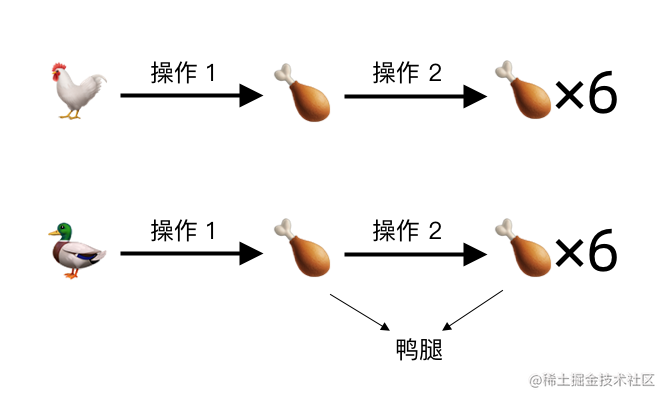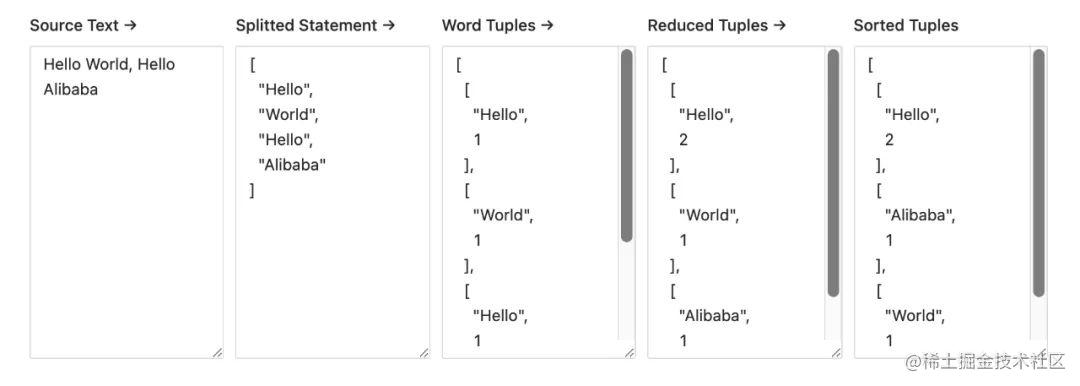我不知道的行为，都是参数

• 发送 Ajax 请求需要知道以下信息：useRequest

• 该请求是否正在等待返回：加载中状态

• 该请求是否成功返回：成功状态

• 该请求是否需要手动触发：触发条件

• 若该请求为自动触发，则触发条件有哪些：依赖刷新

• 该请求是否需要被缓存：缓存条件、缓存状态

• 有一系列涉及到异步操作的行为，为了降低系统的并发量，需要将它们自动进入队列并进行串行执行：runTrack

• 在行为定义的地方希望能做到无感知

• 执行方也不需要感知到队列的存在

• ……

fetchPosts(123)

// ...

其实也没那么深奥的 PLT / 数学概念 —— codata

S=πr^2

• S为圆的面积，也就是这条公式需要求的值；

• \pi为圆周率，作为一个常用无理数，它在不同的场景中会有不同的精度近似值，比如我们在学习的时候一般会使用 3.14 作为一个便于手算的近似值使用，而在对精度要求更高的场景中则可能需要用到小数点后 N 位；

• 然而实际上我们应该把\pi看做圆周率计算算法在不同的近似精度下的结果
• r为圆的半径，而对于这条公式本身来说，r也是一个未知数。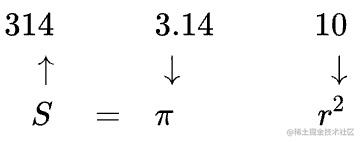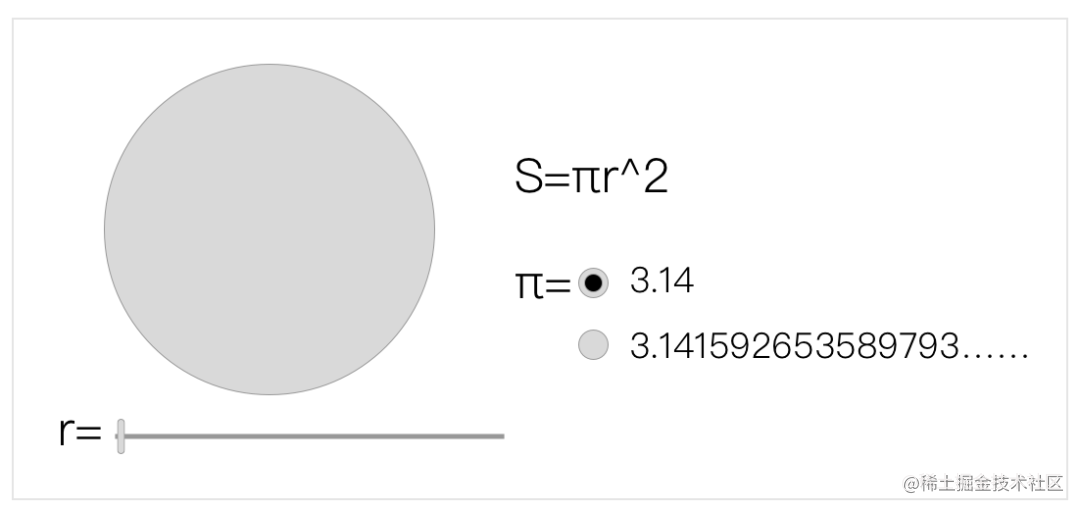1. 一个可以改变圆半径r的滑动条

2. 一个可以改变圆周率\pi所使用近似值的单选框

3. 一个根据所选半径以显示大小的圆形

4. 一个展示圆形面积的字符串

import { useState, useMemo } from 'react'

function App() {
const [r, setR] = useState(10)
const [PI, setPI] = useState(3.14)

const S = useMemo(() => PI * Math.pow(r, 2), [PI, r])
const circleCSS = useMemo(() => ({
width: r * 2,
height: r * 2,
}), [r])
}

Compose & Visit

Render = F\text{<}\pi,r,S,\text{circleCSS}\text{>}

Render = F\text{<}\pi,r,F\text{<}\pi,r\text{>},F\text{<}r\text{>}\text{>}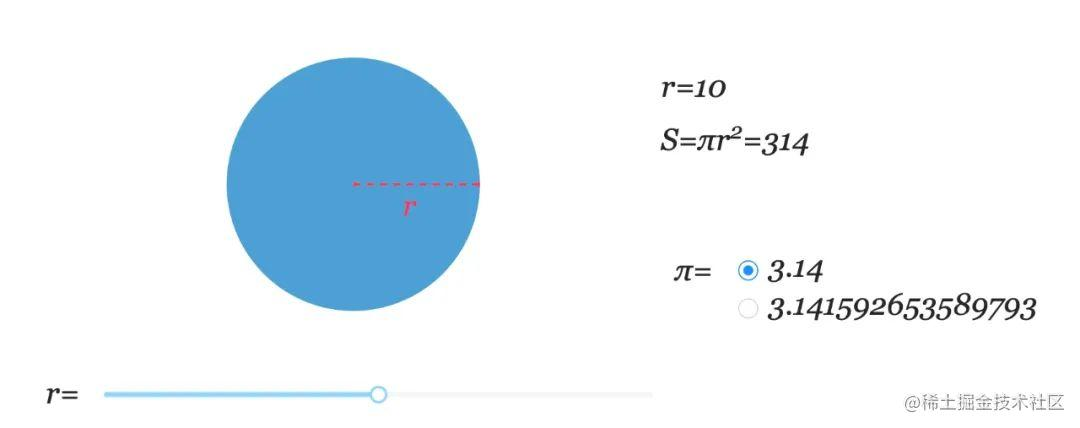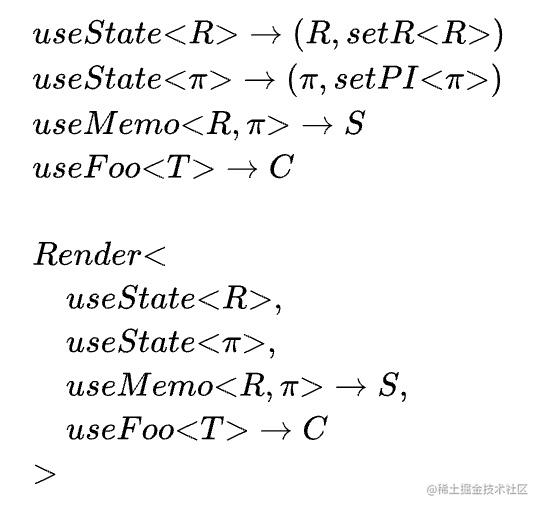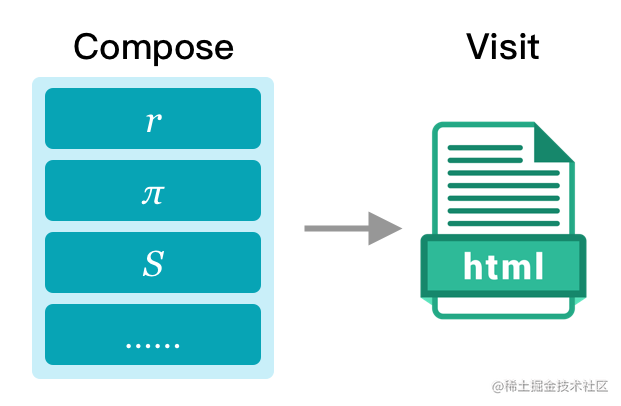React Hooks 与 Vue Composition API

import Vue from "vue"
import VueCompositionAPI, { ref } from "@vue/composition-api"
import Antd from "ant-design-vue"

Vue.use(VueCompositionAPI)
Vue.use(Antd)

const template = 
<a-row id="app">
<a-col :span="24">
<h3>{{count}}</h3>
</a-col>
<a-col :span="12">
<a-button @click="() => incr()">INCR</a-button>
</a-col>
<a-col :span="12">
<a-button @click="() => decr()">DECR</a-button>
</a-col>
</a-row>

new Vue({
name: "App",

template,

setup(props) {
const count = ref(0)
const incr = (increment = 1) => (count.value += increment)
const decr = (decrement = 1) => (count.value -= decrement)

return {
count,
incr,
decr
}
}
}).\$mount("#app")

Play together?

import React from "react"
import { defineComponent, ref } from "reactivue"
import { Row, Col, Button } from "antd"

const Counter = defineComponent(
// Setup
() => {
const count = ref(0)
const incr = (increment = 1) => (count.value += increment)
const decr = (decrement = 1) => (count.value -= decrement)

return {
count,
incr,
decr
}
},
// Render
({ count, incr, decr }: any) => (
<Row style={{ width: 150, textAlign: "center" }} className="App">
<Col span={24}>
<h3>{count}</h3>
</Col>
<Col span={12}>
<Button onClick={() => incr()}>INCR</Button>
</Col>
<Col span={12}>
<Button onClick={() => decr()}>DECR</Button>
</Col>
</Row>
)
)